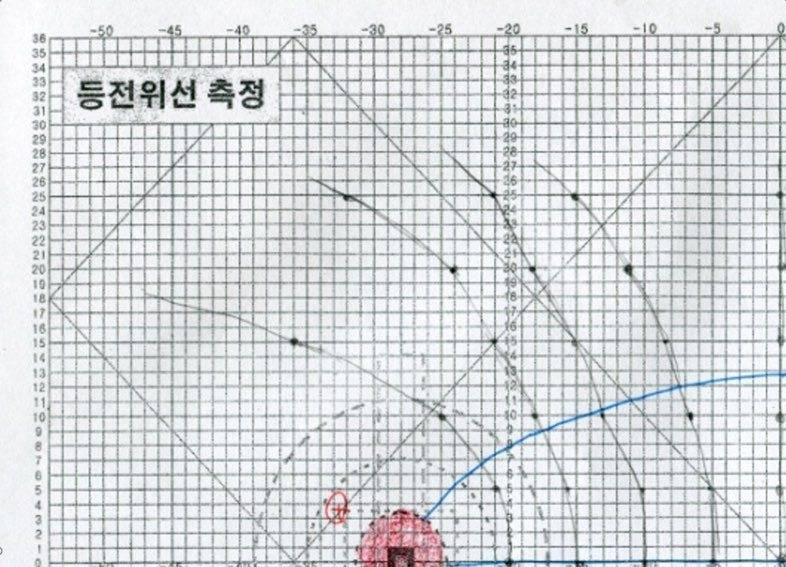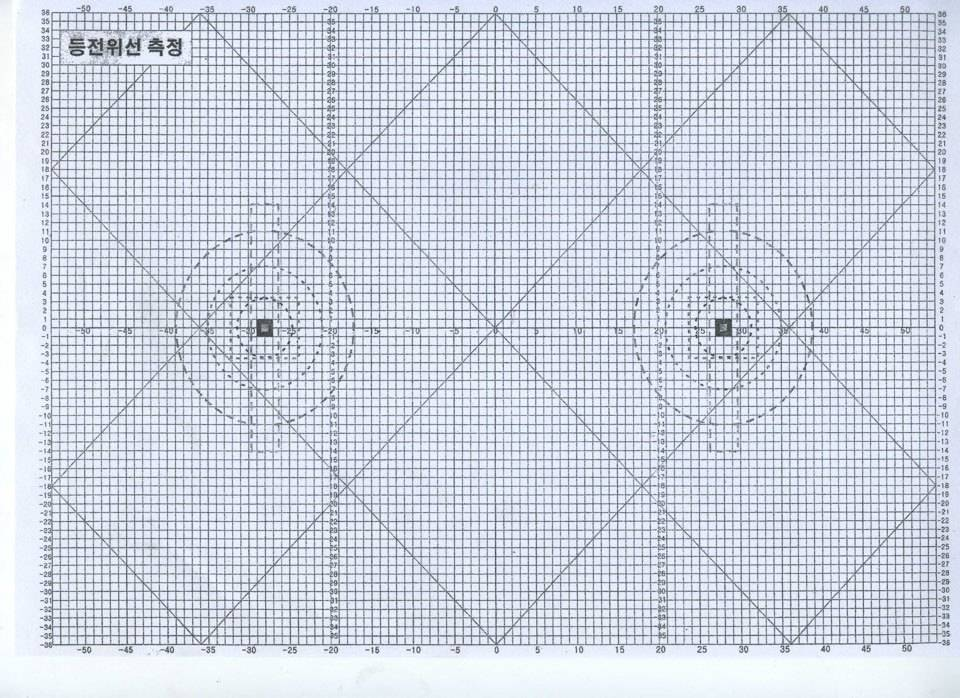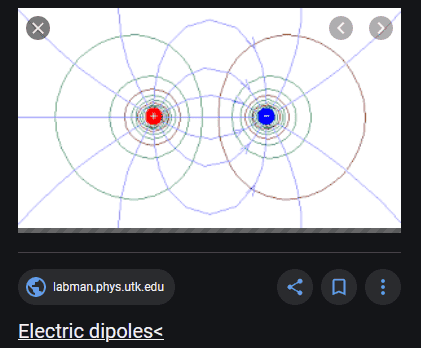Mimyo
Homework Statement:
complete this equipotential line.
Relevant Equations:
.This is the second quadrant of the equipotential line. I think this would be symmetry but I'm not sure what to do.
Is this going to be the symmetry of both the x-axis and y-axis and the symmetry of the y-axis is ( - )?

Sorry for the bad English! feel free to leave comment if you can't understand the question, thank you!

Last edited by a moderator:

Mentor
Homework Statement:: complete this equipotential line.
Relevant Equations:: .

View attachment 270056

This is the second quadrant of the equipotential line. I think this would be symmetry but I'm not sure what to do.
Is this going to be the symmetry of both the x-axis and y-axis and the symmetry of the y-axis is ( - )?

Sorry for the bad English! feel free to leave comment if you can't understand the question, thank you!
Welcome to PhysicsForums.The problem statement and the graph/drawing are very confusing. What is the full problem statement? What is at the origin? Charge? Current? A point of something or a distribution of line charge or something?

And what did the blank diagram look like before you started trying to draw lines on it? Were the long straight lines at angles there before, or did you draw those with a ruler? Were only the dotted lines in arcs on the drawing before, and all of the rest of the lines are yours?

•Mimyo
Mimyo
Welcome to PhysicsForums.The problem statement and the graph/drawing are very confusing. What is the full problem statement? What is at the origin? Charge? Current? A point of something or a distribution of line charge or something?

And what did the blank diagram look like before you started trying to draw lines on it? Were the long straight lines at angles there before, or did you draw those with a ruler? Were only the dotted lines in arcs on the drawing before, and all of the rest of the lines are yours?
Professor just gave me the picture, since we can't do the experiment due to COVID 19. So this is the result of the "equipotential line experiment". The picture I posted is the 1/4 of the result and the original one (That I have to complete) is below. Line of electric force flows from + to -, and you can see the ( + ) in the picture.So, I haven't drawn any lines. According to his advice, I have to symmetry to x or y-axis and connected dots going to be the equipotential line. After that, I have to symmetry the equipotential line again.

Thanks for helping me T_T

Mentor
Okay, thanks. That helps a lot. So there are +/- charges on the x-axis at +/- 28 (whatever units), right?

Probably the Equipotential lines look a lot like the diagram below. But what equation would you use to calculate the E-field at any point as the sum of the contributions from each of the two charges? And how does that relate to the equation for the potential generated from that vector E-field?